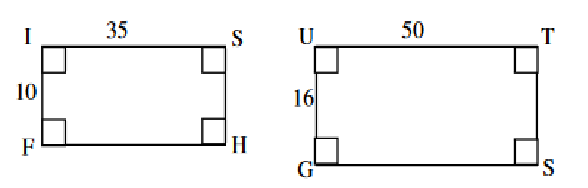GEOB - Scale Factor
Period *
This is THE HARDEST question. Do your best. Describe the relationship between these two triangles *
1 point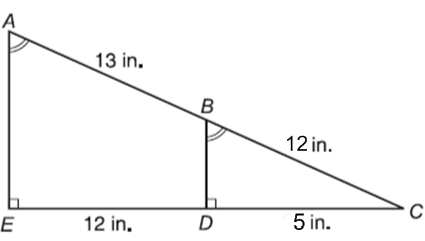What is the scale factor of the quadrilaterals? Remember all you need is to compare CORRESPONDING SIDES AND REDUCE *
1 point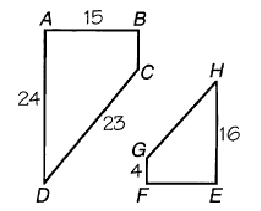Identify the relationship *
1 point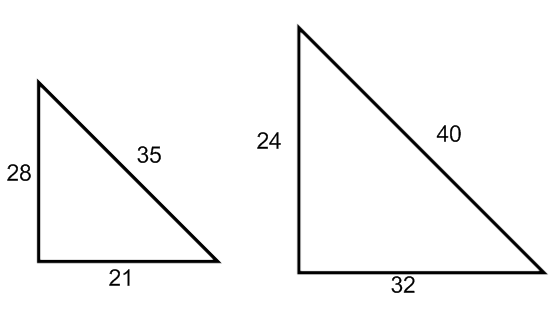These triangles are similar. Identify the scale factor *
1 point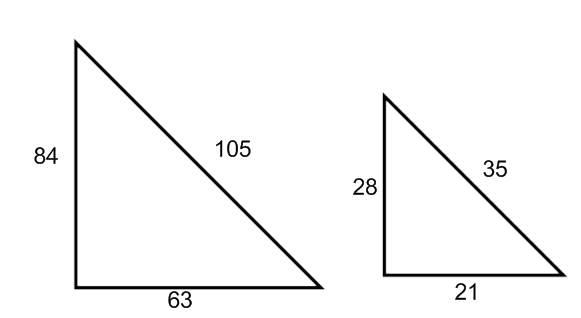Last Name only *
Which option does this picture represent *
1 point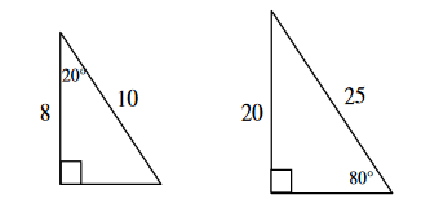Which rectangle is similar to the shaded one? *
1 pointThese two trapezoids are similar. The scale factor is 1/2. Using this scale factor, find the side marked with an x *
1 point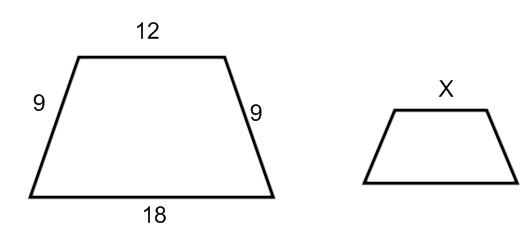Which option represents these polygons *
1 point